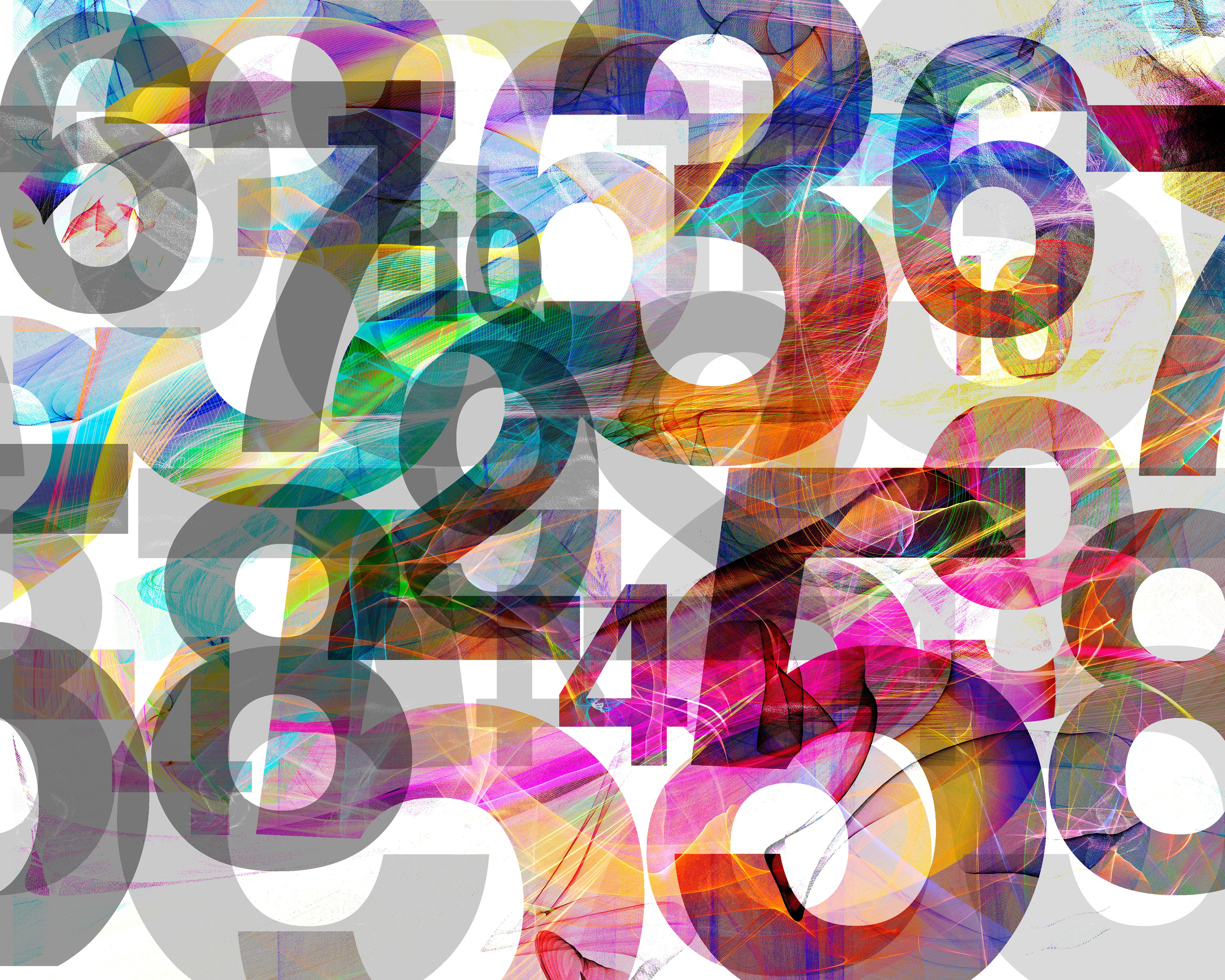Click the link below the picture

.

What is the most bizarre real number that you can imagine? Probably many people think of an irrational number such as pi (π) or Euler’s number. And indeed, such values can be considered “wild.” After all, their decimal representation is infinite, with no digits ever repeating. Even such bonkers-looking numbers, however, together with all the rational numbers, make up only a tiny fraction of the real numbers, or numbers that can appear along a number line. (As a reminder, these are the kinds of numbers that can be used in all manner of familiar measurements, including time, temperature and distance.)

But it turns out that if you happened to pick out a number at random on a number line, you would almost certainly draw a “noncomputable” number. For such values, there is no way to determine them precisely.

The real numbers are made up of the rational and irrational numbers. The rational numbers (that is, numbers that can be written as the fraction pq, where p and q are integers) include the natural numbers (0, 1, 2, 3,…) and the integers (…, –2, –1, 0, 1, 2,…). The rest of the numbers on the number line are irrational numbers. These, too, can be divided into different categories—most of which we can’t even imagine.

.Credit: nagelestock.com/Alamy Stock Photo

.

.

Click the link below for the article:

.

__________________________________________